• ## java实现开根号

万次阅读 2017-08-22 20:46:51
java根号
主要思路
通过牛顿迭代法计算平方根。来吧，还是看代码ba..
    private static double sqrt(double c) {
if (c < 0)
return Double.NaN;

double jingdu = 1e-15;
double t = c;
while (Math.abs(c - t * t) > jingdu)
t = (c / t + t) / 2.0;// 迭代公式
return t;
}
改进算法。。。
    public static double sqrt(double m) {
double i = 0;
int k = 0;
while ((i * i) <= m)
i += 0.1;
for (int j = 0; j < 10; j++) {
i = (m / i + i) / 2;
}
return i;
}
展开全文算法
• java实现开根号的运算 如果某个数字正好可以开根号为2个整数，例如1,4,9等，那就很简单了。 如果某个数字不可以正好开根号为2个整数，而且要保留几位精度，例如：2,3,5等，我们该怎么办呢？？？？？ 首先我们可以...
今天我们来看一下这道题，java如何实现开根号的运算。大家都知道，java,c++,python等都有直接的函数可以给我们用，但是面试官这个时候让我们手撕源码，这个时候怎么做呢，来，不要慌！！！如果某个数字正好可以开根号为2个整数，例如1,4,9等，那就很简单了。
如果某个数字不可以正好开根号为2个整数，而且要保留几位精度，例如：2,3,5等，我们该怎么办呢？？？？？
首先我们可以把这个数字分成整数部分和小数部分，分别计算。
例如√5≈2.236  我们可以先算出整数部分为2，然后在根据保留几位精度，去计算小数部分。依次计算十分位、百分位和千分位等，然后把整数位+十分位+百分位+千分位+。。。，结果就是我们想要的结果了。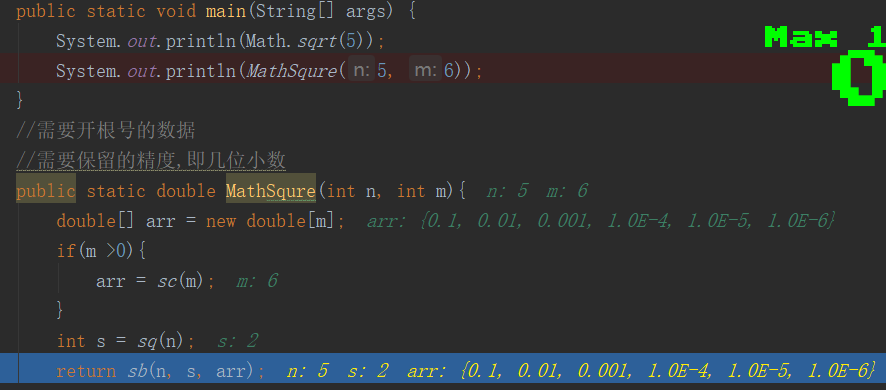来看代码
import java.math.BigDecimal;

/**
* @Auther: liuhaidong
* Data: 2020/4/21 0021、20:55
* Description:
* @version: 1.0
*/
public class Test6 {
public static void main(String[] args) {
System.out.println(Math.sqrt(5));
System.out.println(MathSqure(5, 6));
}
//需要开根号的数据
//需要保留的精度,即几位小数
public static double MathSqure(int n, int m){
double[] arr = new double[m];
if(m >0){
arr = sc(m);
}
int s = sq(n);
return sb(n, s, arr);
}
/**
* 计算要保留几位小数
* @param m
* @return
*/
public static double[] sc(int m){
double[] arr = new double[m];
int num = 0;
while(num != m){
double f = 1;
for(int i=0;i<=num;i++){
f = f*10;
}
arr[num] = 1/f;
num++;
}
return arr;
}
/**
* 计算整数位
* @param n
* @return
*/
public static int sq(int n){
if( n == 1){
return 1;
}
int tmp = 0;
for(int i=1;i<=n/2+1;i++){
if(i*i == n){
tmp = i;
break;
}
if(i*i > n){
tmp = i-1;
break;
}
}
return tmp;
}

/**
* 开根号
* @param n
* @param j
* @param arr
* @return
*/
public static double sb(int n, double j, double[] arr){
double tmp = j;
for(int p=0;p<arr.length;p++){
if(p>0){
j = tmp;//计算过后的值（整数位+小数位的和，赋值给j，下面继续运算）
}
for(int i=1;i<=9;i++){//小数位只有九位{0.1,0.2,0.3,0.4,0.5,0.6,0.7,0.8,0.9}
tmp = i*arr[p]+j;//i*arr[p],相当于每次加0.1,0.2 ...
if(tmp*tmp == n){
return tmp;
}
if(tmp*tmp >n){
//避免丢失精度
BigDecimal c1 = new BigDecimal(Double.toString(tmp));
BigDecimal c2 = new BigDecimal(Double.toString(arr[p]));
tmp = c1.subtract(c2).doubleValue();
break;
}
}
}
return tmp;
}
}

思路继续讲解。
小于2.3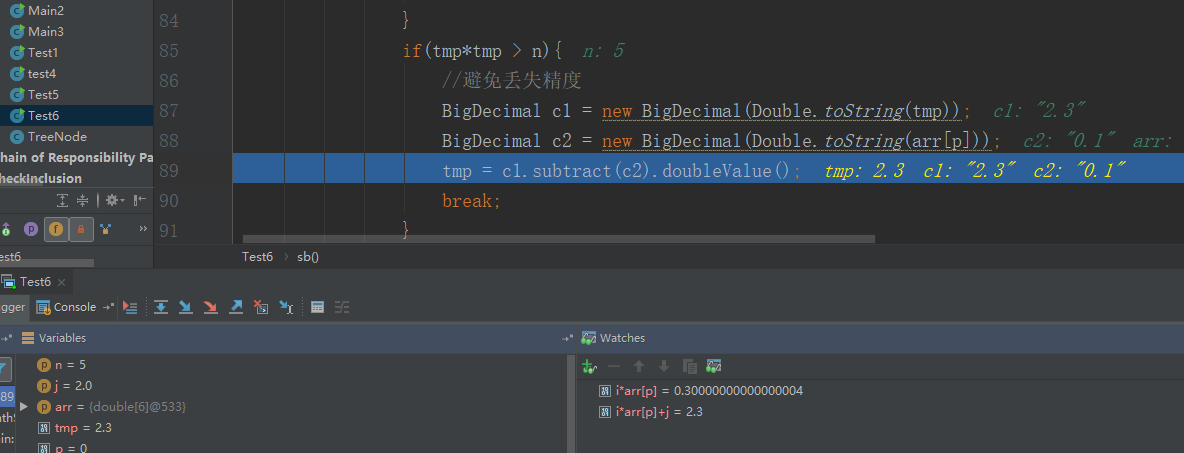把2.2记录在这里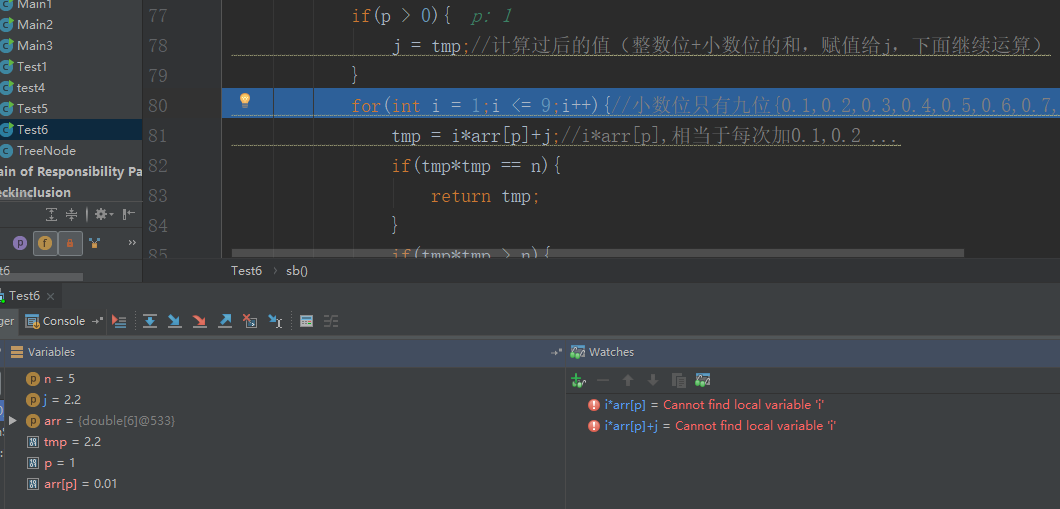小于2.24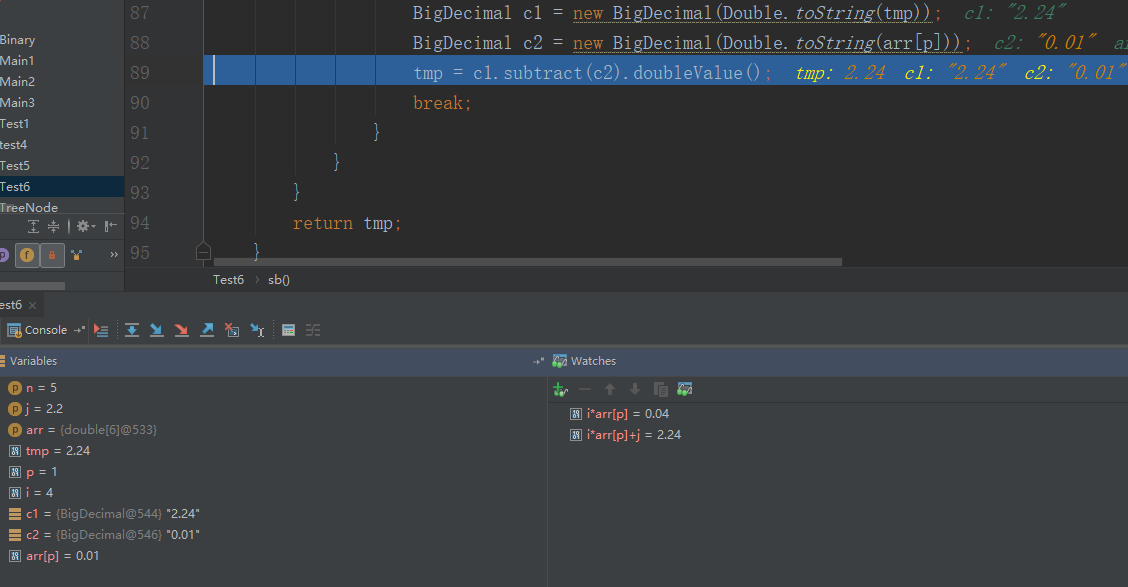记录2.23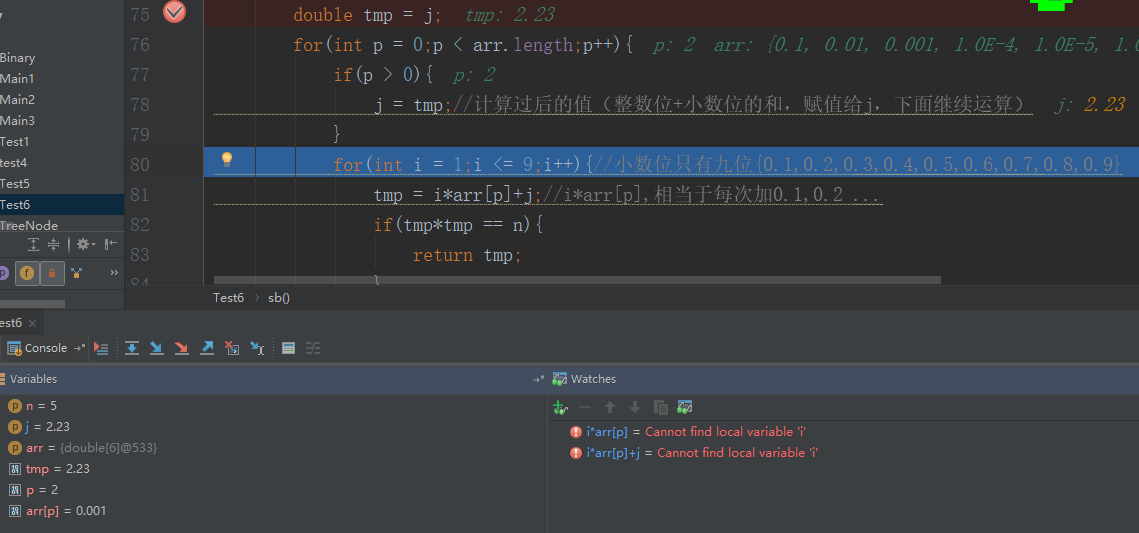依次这样做。本公众号分享自己从程序员小白到经历春招秋招斩获10几个offer的面试笔试经验，其中包括【Java】、【操作系统】、【计算机网络】、【设计模式】、【数据结构与算法】、【大厂面经】、【数据库】期待你加入！！！
1.计算机网络----三次握手四次挥手 2.梦想成真-----项目自我介绍 3.你们要的设计模式来了 4.震惊！来看《这份程序员面试手册》！！！ 5.一字一句教你面试“个人简介” 6.接近30场面试分享 7.你们要的免费书来了
展开全文• 首先根号2一定在0到2之间， 找出0和2的中点1，1的平方还是1，小于2，所以根号2一定在1到2之间； 找出1和2的中点1.5，1.5的平方是2.25，大于2，所以根号2一定在1到1.5之间； 找出1和1.5的中点1.25，1.25的平方是1....
二分法 首先根号2一定在0到2之间， 找出0和2的中点1，1的平方还是1，小于2，所以根号2一定在1到2之间； 找出1和2的中点1.5，1.5的平方是2.25，大于2，所以根号2一定在1到1.5之间； 找出1和1.5的中点1.25，1.25的平方是1.5625，小于2，所以根号2一定在1.25到1.5之间； 以此类推，直到找到小数点后第k位。 这种方法很简单，容易想到，但就是有些麻烦。代码如下： public static double gen(int k) {         double left = 0;         double right = 2;         double middle = (left+right)/2;         while(String.valueOf(middle).length()<=k+1) {             if(Math.pow(middle, 2)<=2) {                 left = middle;             }             if(Math.pow(middle, 2)>2) {                 right = middle;             }             middle = (left + right)/2;         }         return middle;     } }
展开全文算法
• ## Java实现开根号

千次阅读 2018-09-30 02:21:47
Java实现开根号 二分法 static float sqr(float x){ float max = x; float min = 0; float mid=(min+max)/2; while (true){ if (Math.abs(x-mid*mid)&amp;lt;0.002) return mid;...
Java实现开根号
二分法
   static float sqr(float x){
float max = x;
float min = 0;
float mid=(min+max)/2;
while (true){
if (Math.abs(x-mid*mid)<0.002) return mid;

if(mid*mid>x)
{
max=mid;
}
else if(mid*mid<x)
{
min=mid;
}

mid=(min+max)/2;
}

}

牛顿法
牛顿迭代法（Newton’s method）又称为牛顿-拉夫逊方法（Newton-Raphson method），它是牛顿在17世纪提出的一种在实数域和复数域上近似求解方程的方法。多数方程不存在求根公式，因此求精确根非常困难，甚至不可能，从而寻找方程的近似根就显得特别重要。方法使用函数f(x)的泰勒级数的前面几项来寻找方程f(x) = 0的根。牛顿迭代法是求方程根的重要方法之一，其最大优点是在方程f(x) = 0的单根附近具有平方收敛，而且该法还可以用来求方程的重根、复根。另外该方法广泛用于计算机编程中。
设r是f(x) = 0的根，选取x0作为r初始近似值，过点（x0,f(x0)）做曲线y = f(x)的切线L，L的方程为y = f(x0)+f’(x0)(x-x0)，求出L与x轴交点的横坐标 x1 = x0-f(x0)/f’(x0)，称x1为r的一次近似值。
过点（x1,f(x1)）做曲线y = f(x)的切线，并求该切线与x轴交点的横坐标 x2 = x1-f(x1)/f’(x1)，称x2为r的二次近似值。重复以上过程，得r的近似值序列，其中x(n+1)=x(n)－f(x(n))/f’(x(n))，称为r的n+1次近似值，上式称为牛顿迭代公式。
    static float Newton_sqr(float x){
float k = 1;
while (Math.abs(k*k-x)>1e-5){
k=(k+x/k)/2;
}
return k;
}

参考
https://blog.csdn.net/huaqianmian/article/details/51279627?utm_source=copy
展开全文• ## Java 计算根号2

千次阅读 2018-12-13 10:41:35
计算根号2有两种方法 1.二分查找 2.牛顿法 public static void main(String[] args) { double sqrNum = getSqrNum(2); System.out.println(sqrNum); double sqrNum2 = getSqrNum2(2); System.out....
• 平方，开根号java中是很简单的，Math.sqrt(double n)或者 Math.pow(double a, double b)，求a的b次方。但是我们可以自己想想，这些方法到底是怎么实现的。 就拿开根号来解释，它有两种方法，二分法和牛顿迭代法。...
• 根号运算是数学的基本运算，对于无理数的求解，我们可以使用两边取值，不断逼近的方法进行求解。现要求你编程实现根号3的求解。 输入格式 　无输入 输出格式 　输出根号3的求解结果 样例输入 无 样例输出 无 数据...算法
• ## java实现开根号算法

千次阅读 2018-05-03 00:04:00
public static void main(String[] args) { long start = System.currentTimeMillis(); double target=9876543212345d; double result =sqrt(target); System.out.printl...
• 在做数学题的时候我们是要保留根号的。这里提供带根号的开平方方法 //开平方，数组第一个放平方根前面的系数，第二个放根号里面无法开方的数 public static int[] mySqrt(int n) { int ints[]=new int; for...
• 读《西方哲学史》古希腊早期数学与天文学一章，看到一个有趣的求解根号2的方法，之前未曾见过。   思路如下：构造一个数对序列，初始值为（1，1）， 然后对该数对依照如下规则进行演化： 下一个数对中的第一个...
• Java中的运算符Java 语言中常用的运算符Ø 算术运算符Ø 赋值运算符Ø 比较运算符Ø 逻辑运算符Ø位运算符Ø 条件运算符（三元运算符）算数运算符算术运算符主要用于进行基本的算术运算，如加法、减法、乘法、除法等...
• 需求：在不借助系统库的情况下，编写一个函数，实现开根号的操作，并且保证一定的精度 代码采用了牛顿迭代法以及二分查找法两种方式并分别打印了他们的循环次数以比较优劣： /** * 实现一个函数，完成对v开根号的...
• import java.util.*; public class Solution { /** * 根据平方数的性质——连续n个奇数相加的结果一定是平方数。 * 如：9=1+3+5； * 16=1+3+5+7； * 所以，不断的进行奇数相加，并判断x大小即可 有几个奇数 ...
• package classifier; public class getSqrt { public static void main(String[] args){ double a = 15; System.out.println(Math.sqrt(a)); System.out.println(Math.floor(Math.sqr...
• Java语言编程计算根号2，要求不能调用math中的函数，要用循环来编写开发语言
• Java利用二分法开根号 package com.sankuai.ead.urm.dao.ext; /** * 利用二分法开根号 */ public class Test { static double r; public static void sqrt(double number) { process(number, 0, number); } ...二分法
• ## Java异常处理

千次阅读 2015-10-02 11:15:37
你觉得自己是一个Java专家吗？是否肯定自己已经全面掌握了Java的异常处理机制？在下面这段代码中，你能够迅速找出异常处理的六个问题吗？ 1 OutputStreamWriter out = ... 2 java.sql.Connection conn = ... 3 ...Exception 异常处理
• public class Math {public static void main(String[] args) {double a=0.0001;double result ;for (double i=2.0;i<=5.0;i+=a) {result=i*i;double b=5.0-a;if ((result>=b)&&resul...
• Problem Description 飞飞特别喜欢平方数，可是他数学并不好... double w=java.lang.Math.sqrt(j);  int e = (int)w;  if(e*e==j)p+=j;  }  System.out.println(p);  }  input.close();  } }
• ## java大数开根号问题

千次阅读 2017-11-05 10:17:04
import java.util.*; import java.math.*; public class Main{ static BigInteger cal(BigInteger x){ BigInteger l = BigInteger.ONE ; BigInteger r = x ; BigInteger temp = BigInte
• 质数 分析: 质数有什么特点？ 大于1,只能被1和本身整除的,再没有其他因数的数 方法1: n<=3时，质数有2和3 当n>3时，质数无法被比它小的数整除 public static boolean ... return n &...
• import java.awt.; import java.awt.event.; public class caculator extends JFrame implements ActionListener { private static final long serialVersionUID = 1L; private boolean dotExist, operated, ......

# java根号java 订阅